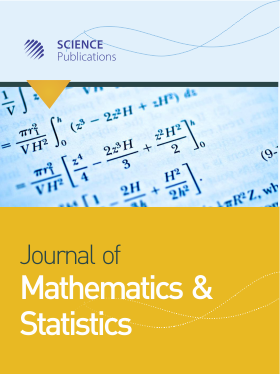Frequency: Continuous
ISSN: 1549-3644 (Print)
ISSN: 1558-6359 (Online)
Research Article Open Access

# Half-bounded Numerical Solution of Singular Integral Equations with Cauchy Kernel

M. Abdulkawi1, Z. K. Eshkuvatov1 and N.M.A. Nik Long2
• 1 ,
• 2 , Afganistan
Journal of Mathematics and Statistics
Volume 7 No. 1, 2011, 81-85

DOI:

Submitted On: 12 November 2010 Published On: 25 March 2011

How to Cite: Abdulkawi, M., Eshkuvatov, Z. K. & Long, N. N. (2011). Half-bounded Numerical Solution of Singular Integral Equations with Cauchy Kernel. Journal of Mathematics and Statistics, 7(1), 81-85. https://doi.org/10.3844/jmssp.2011.81.85

## Abstract

Problem statement: In this study, a numerical solution for singular integral equations of the first kind with Cauchy kernel over the finite segment [-1,1] is presented. The numerical solution is bounded at x =1 and unbounded at x = -1. Approach: The numerical solution is derived by approximating the unknown density function using the weighted Chebyshev polynomials of the fourth kind. Results: The force function is approximated by using the Chebyshev polynomials of the third kind. Conclusion: The exactness of the numerical solution is shown for characteristic equation when the force function is a cubic.

• 1,069 Views